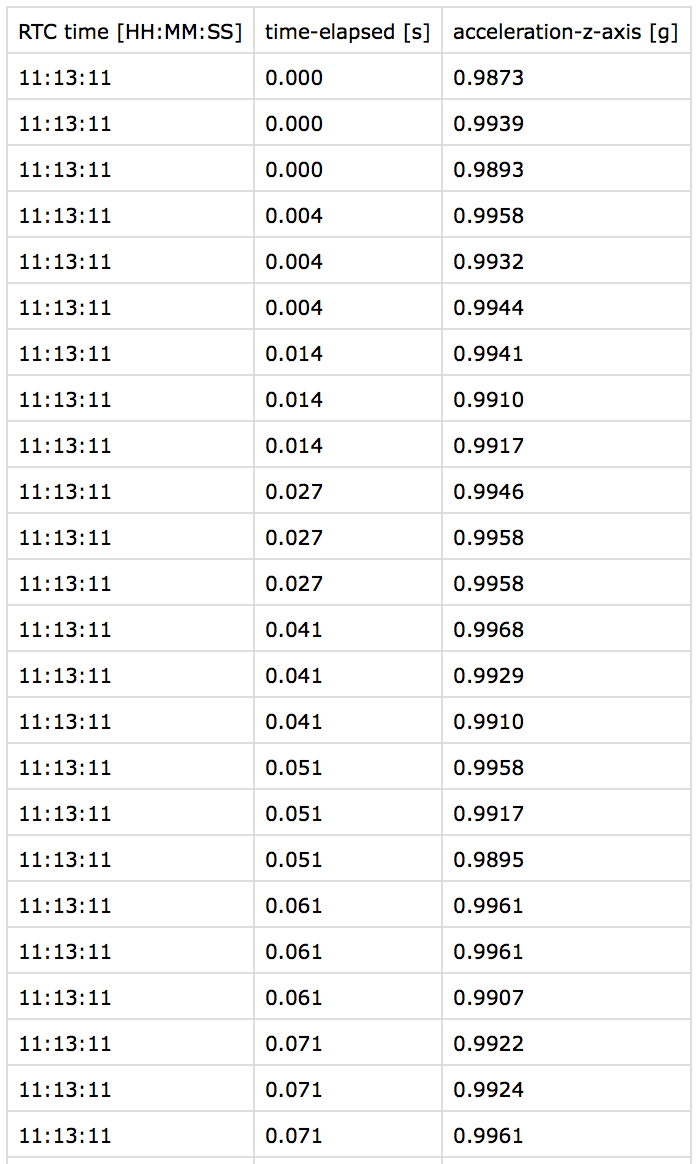Packed acceleration - same timestamps

I am wondering why I get three equal timestamps in a row when recording packed acceleration. Does it mean there is only one data.epochfor the BLE data package?

Here is an excerpt of my implementation:

function calcElapsedTime(epoch, stream) {
if (!('first' in stream)) {
stream.first = epoch;
}
return ((epoch - stream.first) / 1000.0).toFixed(3); // max accuracy 1ms
}

and ...

let onAccDataCallback = (dataPtr) => {
let data = dataPtr.deref();
let elapsedTimeInSec = calcElapsedTime(data.epoch, stream);
// ...
}